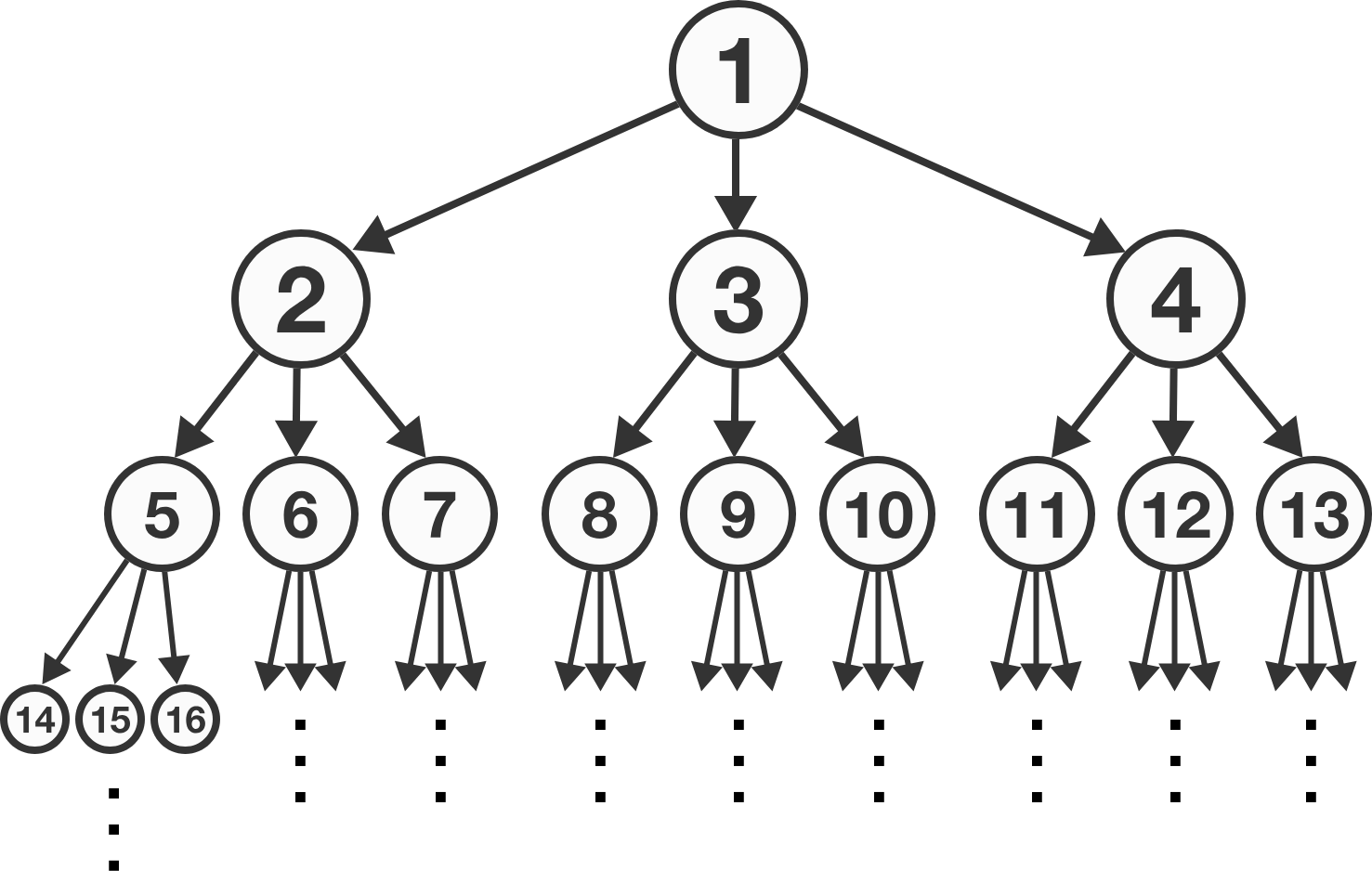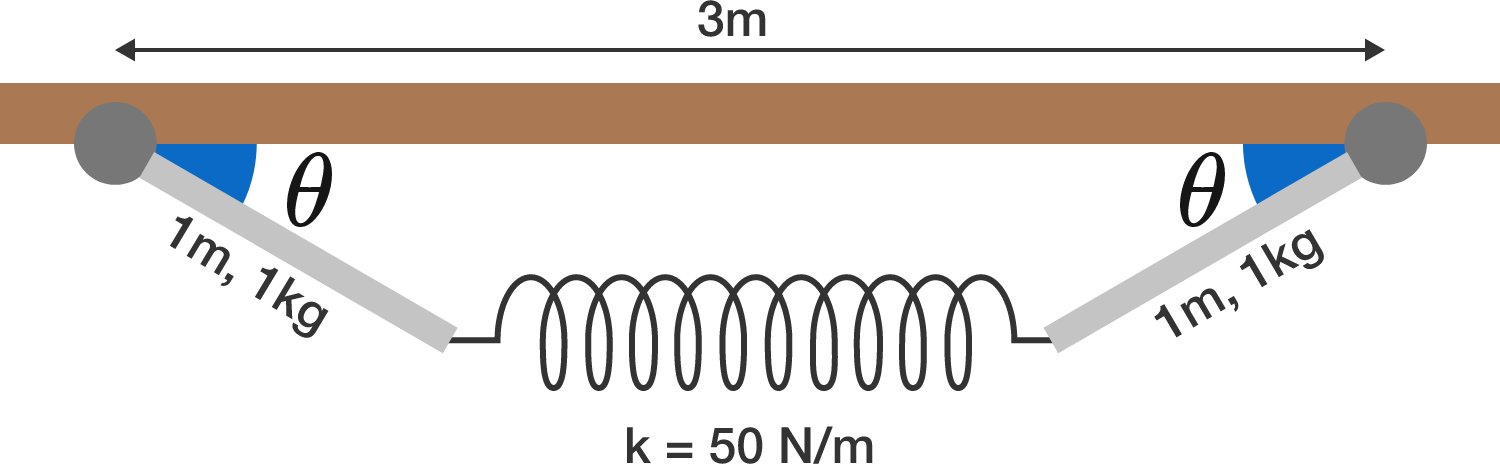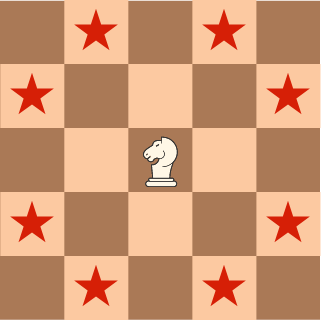# Problems of the Week

Contribute a problem

# 2017-11-13 IntermediateThe pyramid of integers above is constructed in such a way that each "father" has exactly 3 "children": $1 \to 2\ \, 3\ \, 4, \quad 2 \to 5\ \, 6\ \, 7, \quad 3 \to 8\ \, 9\ \, 10, \quad 4 \to 11\ \, 12\ \, 13, \quad 5 \to 14\ \, 15\ \, 16,\quad ......$ What number is the father of 2017?

$1,\ 2,\ 3,\ 4,\ 5$

These five numbers are written on a whiteboard. You start with a score of 0. You pick two numbers, add their positive differences to your score, and erase your choice of one of the two numbers. This process is repeated until there is only one number left on the whiteboard.

What is the highest score you can get?

For example, if you first pick 1 and 2 and erase 2, your score becomes $2-1=1,$ and only $1,3,4,5$ are left on the whiteboard.

Two identical, massive rods are hinged at one end and connected together by a massless ideal spring.

If the system is in static equilibrium, what is the angle $\theta$ (in degrees)?


Details and Assumptions:

• Each rod is $1 \text{ m}$ long and has a mass of $1 \text{ kg}.$
• The spring constant is $k = 50 \text{ N/m}$, and the spring's unstretched length is $1 \text{ m}.$
• The two hinges are $3 \text{ m}$ apart horizontally.
• The strength of gravity is $10 \text{ m/s}^2$ in the downward, vertical direction.A recursive sequence is defined as $a_0=\pi$ and $a_n=\log_{a_{n-1}}{27}.$

Given that $\displaystyle\lim_{n\to\infty}{a_n}$ exists, what is $\displaystyle\lim_{n\to\infty}{a_n}?$

Bonus: For what values of $a_0$ does $\displaystyle\lim_{n\to\infty}{a_n}$ exist?

This problem is part of the new Brilliant.org Open Problems Group. The end goal for each open problem is to find a solution, and maybe publish it if it's a nice enough result! Even if we don't make it all the way there, we can have fun exploring unsolved problems and doing real research. This problem is related to an unsolved open problem, which you can read about here.

Place an equal number of white knights and black knights on a square board (of any size) such that

• each white knight is attacking 2 other white knights and 2 black knights;
• each black knight is attacking 2 other black knights and 2 white knights.

What is the side length of the smallest square board on which this is possible?Note: A chess knight moves in an "L" shape either 1 square vertically and 2 squares horizontally or 2 squares vertically and 1 square horizontally, as indicated by the stars above.

×